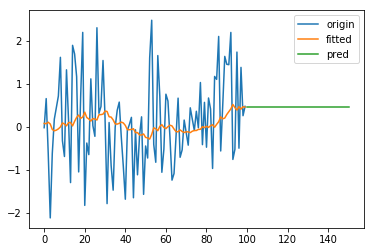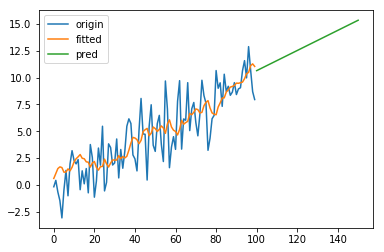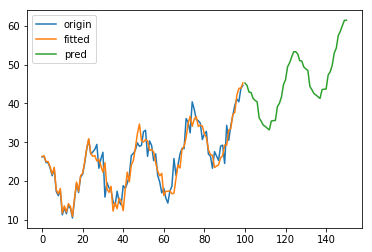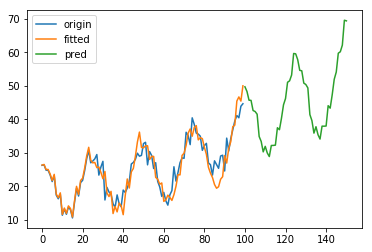## 1. 一次指数平滑

Forecast equation: $\hat{y}_{t+h|t} = l_t$
Smoothing equantion: $l_t = \alpha y_t + (1-\alpha)l_{t-1}$

$(\alpha^*, l_0^*) = \min\limits_{(\alpha, l_0)}\sum\limits_{t=1}^T\epsilon_t^2 = \min\limits_{(\alpha, l_0)}\sum\limits_{t=1}^T\left(y_t - \hat{y}_{t|t-1}\right)^2$## 2. 二次指数平滑

### 2.1 Holt’s linear trend method

Holt 扩展了简单指数平滑，使其可以用来预测带有趋势的时间序列。直观地看，就是对平滑值的一阶差分（可以理解为斜率）也作一次平滑。模型的预测结果是一条斜率不为0的直线。模型形如：

Forecast equation: $\hat{y}_{t+h|t} = l_t + hb_t$
Level equation: $l_t = \alpha y_t + (1-\alpha)(l_{t-1} + b_{t-1})$
Trend equation: $b_t = \beta(l_t - l_{t-1}) + (1-\beta)b_{t-1}$### 2.2 Damped trend methods

Holt’s linear trend method 得到的预测结果是一条直线，即认为未来的趋势是固定的。对于短期有趋势、长期趋于稳定的序列，可以引入一个阻尼系数 $0<\phi<1$，将模型改写为

Forecast equation: $\hat{y}_{t+h|t} = l_t + (\phi + \phi^2 + \cdots +\phi^h)b_t$
Level equation: $l_t = \alpha y_t + (1-\alpha)(l_{t-1} + \phi b_{t-1})$
Trend equation: $b_t = \beta(l_t - l_{t-1}) + (1-\beta)\phi b_{t-1}$

## 3. 三次指数平滑

Forecast equation: $\hat{y}_{t+h|t} = l_t + hb_t + s_{t+h-m(k+1)}$
Level equation: $l_t = \alpha(y_t - s_{t-m}) + (1-\alpha)(l_{t-1} + b_{t-1})$
Trend equation: $b_t = \beta(l_t - l_{t-1}) + (1-\beta)b_{t-1}$
Seasonal equation: $s_t = \gamma(y_t - l_{t-1} - b_{t-1}) + (1-\gamma)s_{t-m}$### 3.2 Holt-Winters’ multiplicative method

Forecast equation: $\hat{y}_{t+h|t} = (l_t + hb_t)s_{t+h-m(k+1)}$
Level equation: $l_t = \alpha\dfrac{y_t}{s_{t-m}} + (1-\alpha)(l_{t-1} + b_{t-1})$
Trend equation: $b_t = \beta(l_t - l_{t-1}) + (1-\beta)b_{t-1}$
Seasonal equation: $s_t = \gamma\dfrac{y_t}{(l_{t-1} + b_{t-1})} + (1-\gamma)s_{t-m}$### 3.3 Holt-Winters’ damped method

Holt-Winters’ 模型的趋势部分同样可以引入阻尼系数 $\phi$，这里不再赘述。

## 4. 参数优化和模型选择

AIC 即 Akaike information criterion， 定义为

$AIC = 2k - 2\ln L(\theta)$

BIC 即 Bayesian information criterion，定义为

$BIC = k\ln n - 2\ln L(\theta)$

## 5. 与 ARIMA 的关系

$l_t = \alpha y_t + (1-\alpha)l_{t-1}$ 可以改写为

$\hat y_{t+1} = \alpha y_t + (1-\alpha)\hat y_t = \alpha y_t +(1-\alpha)(y_t-\epsilon_t) = y_t - (1-\alpha)\epsilon_t$

$\hat y_t = y_{t-1} -(1-\alpha) \epsilon_{t-1}$

$y_t = \hat y_t + \epsilon_t = y_{t-1} + \epsilon_t - (1-\alpha)\epsilon_{t-1}$

$\left(1-\sum\limits_{i=1}^p\phi_iL^i\right)(1-L)^dX_t = \left(1+\sum\limits_{i=1}^q\theta_iL^i\right)\epsilon_t$

$(1-L)X_t = (1 + \theta_1L)\epsilon_t$

$X_t - X_{t-1} = \epsilon_t + \theta_1\epsilon_{t-1}$

$X_t = X_{t-1} + \epsilon_t + \theta_1\epsilon_{t-1}$

$\theta_1=-(1-\alpha)$，则两者等价。

## 参考文献

 Hyndman, Rob J., and George Athanasopoulos. Forecasting: principles and practice. OTexts, 2014.
 Exponential smoothing - Wikipedia
 Introduction to ARIMA models - Duke